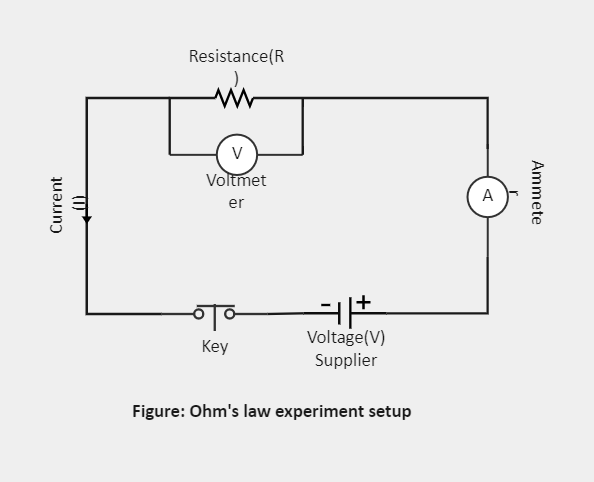Template Community / Ohms Law Circuit Diagram

# Ohms Law Circuit DiagramBlackwood
Published on 2022-03-23
Edit OnlineIntroduction

The current that passes through most substances is directly proportional to the voltage V. Ohm's Law can be viewed as a cause-and-effect relationship, with voltage the cause and current the effect. Ohm's Law shows us that if a conductor is at a constant temperature, the current passing through the conductor is directly proportional to the voltage across it. This indicates that if we plot voltage on the x-axis of a graph and current on the graph's y-axis, we will get a straight line. Let's understand more about it through the Ohms Law Circuit Diagram.

1. Understanding Ohms Law Circuit Diagram

Ohm's Law only holds if the given temperature and the other physical factors remain constant. In certain elements, increasing the current raises the temperature. An example of this is the filament of a light bulb, in which the temperature rises as the current is increased. In this case, Ohm's Law cannot be applied. The Ohms Law Circuit Diagram consists of resistance, voltmeter, key, voltage supplier, and ammeter.

In this circuit, resistance measures the opposition to current flow in an electrical circuit. The main purpose of the resistor is to block the flow of current. Also, they are used to reduce current flow, adjust signal levels, divide voltages, bias active elements, and terminate transmission lines, among other uses.
The voltmeter performs the function of measuring the electrical potential difference between two points in an electric circuit. It also measures either direct or alternating electric current voltages on a scale, usually in volts, millivolts, or kilovolts.
As you know, the ammeter measures either direct or alternating electric current in amperes. Here, an ammeter can measure a wide range of current values because, at high values, only a small portion of the current is directed through the meter mechanism; a shunt in parallel with the meter carries the major portion.

2. How to Create an Ohm's Circuit Diagram using EdrawMax Online?

Creating an Ohm's Circuit Diagram in EdrawMax Online is pretty simple. The free Electrical Circuit maker has several features as you can instantly import the images or icons from your system, Google Drive, or DropBox. Your Electrical Circuit diagram will require additional media content, making it more creative.

Choose a template
EdrawMax Online comes with hundreds of free diagram templates. Select a pre-designed template by entering the Keyword in the "Search" section or exploring different diagram sets. In this case, you will find different types of Electrical Circuits diagrams under the "Electrical Engineering" section under the "Engineering" section. You will find different diagram sets, like Basic Electrical, Circuits and Logic, Industrial Control System, and Systems' Diagram. Alternatively, you can click on "+" in EdrawMax Online canvas to create a diagram of your preference.

As shown in the Ohm's Law Circuit Diagram below, Ohm's Law states that the current flowing through a conductor is directly proportional to the potential difference applied across its ends, provided the temperature and other physical conditions remain unchanged.

Customize the diagram
Customize your Ohm's Circuit diagrams by changing the color or adding more relevant data. Based on your research, you can also add or remove the Electrical Circuit symbols or content accordingly. Since it is about electrical engineering research, you can add more relevant data about different circuit diagrams.

Export & Share
Once your required circuit diagram is completed, you can share it amongst your colleagues or clients using the easy export and share option. You can further export the diagram in multiple formats, like Graphics, JPEG, PDF, or HTML. Also, you can share the designs on different social media platforms, like Facebook, Twitter, LinkedIn, or Line. In simple words, you can export your incredible Ohm's Circuit diagrams to the files you want with just a few clicks.

3. Important Tips to Consider While Creating Ohm's Law Circuit Diagram
It is imperative to take care of some of the points while creating the Ohms Law Circuit Diagram. Let's look at some of them:

Use simplified standard symbols to represent components in the diagram. It will make it easier for users to understand the functionality and components.
Mention the specification of each component along with it, if any.
Try to connect components parallelly, and in series, wherever required.
Cross-check the input and output connections of the components in the circuit

Conclusion

Ohm's Law describes the current flow through a resistance when different electric potentials are applied at each end of the resistance. The main applications of Ohm's Law include determining the voltage, resistance, or current of an electric circuit. It is recommended to use EdrawMax Online to create similar-looking circuit diagrams for your projects. It should be noted here that since these circuit diagrams are the blueprints of the actual working models, ensure that you use the correct symbols provided by EdrawMax Online.
Tag
circuit diagram
electrical plan
Circuit Diagram Collection
Report
2
698PostRecommended Templates